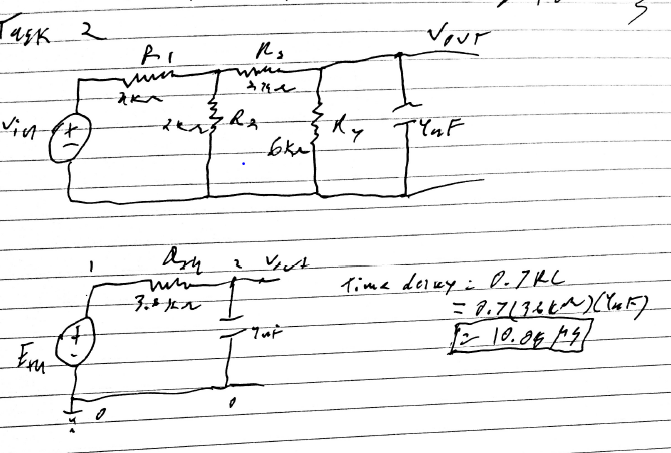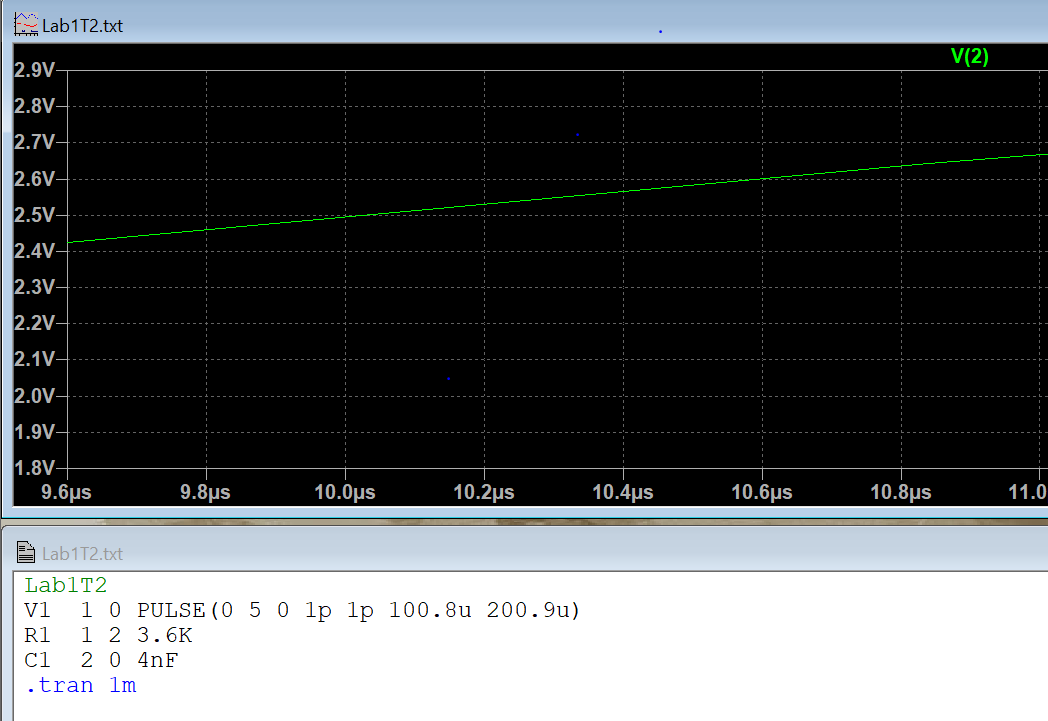ENGR338 Digital Electronics 2021 Spring
Lab 1 - Review of Superposition, Thevenin's Equivalent Circuit, and LTSpice
Name:
Ryan Jeanes
Email: rejeanes@fortlewis.edu

Superposition, Thevenin's Equivalent, and LTSpice

Task 1 - Reviewing Superposition and SpiceFig 1 - Worked by hand to find that Ia=1.4mA, Ib=0.6mA, and Ic=0.8mA

In the LTSpice simulation, the absolute value of the results were the same, but LTSpice had a different frame of reference for the current directions.Fig 2 - LTSpice simulaton showed results as Ia= -1.4mA, Ib= -0.6mA, and Ic= -0.8mA, confirming the work by hand.

Task 2 - Review Thevenin's Equivalent Circuit and simulate in LTSpiceFig 3 - Hand calculation using Thevenin's Equivalent circuit to calculate a time delay of 10.08 microsecondsFig 4 - LTSpice simulaton gave a time delay of approximately 10 microseconds, which corresponds to the calculation by hand of 10.08 microseconds